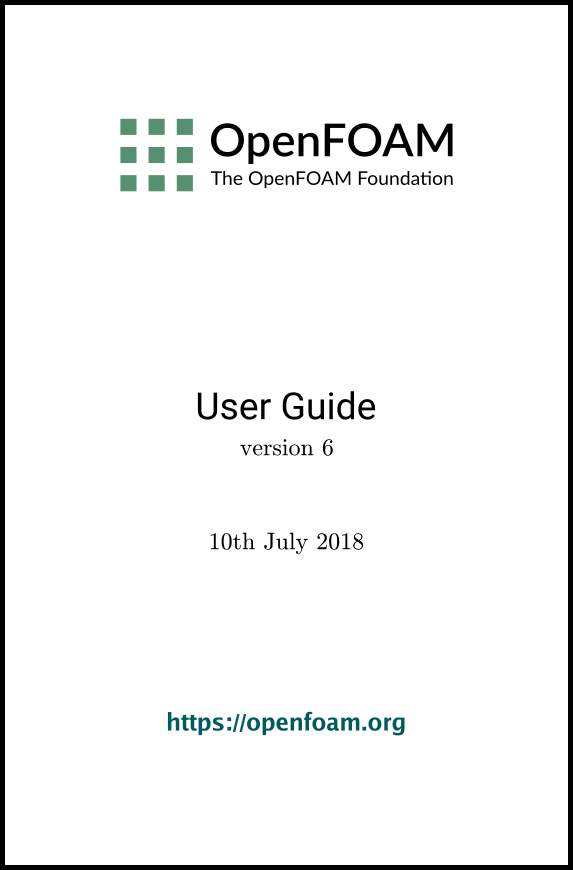## 4.5 Solution and algorithm control

The equation solvers, tolerances and algorithms are controlled from the fvSolution dictionary in the system directory. Below is an example set of entries from the fvSolution dictionary required for the icoFoam solver.

17
18  solvers
19  {
20      p
21      {
22          solver          PCG;
23          preconditioner  DIC;
24          tolerance       1e-06;
25          relTol          0.05;
26      }
27
28      pFinal
29      {
30          \$p;
31          relTol          0;
32      }
33
34      U
35      {
36          solver          smoothSolver;
37          smoother        symGaussSeidel;
38          tolerance       1e-05;
39          relTol          0;
40      }
41  }
42
43  PISO
44  {
45      nCorrectors     2;
46      nNonOrthogonalCorrectors 0;
47      pRefCell        0;
48      pRefValue       0;
49  }
50
51
52  // ************************************************************************* //

fvSolution contains a set of subdictionaries, described in the remainder of this section that includes: solvers; relaxationFactors; and, PISO, SIMPLE or PIMPLE.

### 4.5.1 Linear solver control

The first sub-dictionary in our example is solvers. It specifies each linear-solver that is used for each discretised equation; here, the term linear-solver refers to the method of number-crunching to solve a matrix equation, as opposed to an application solver, such as simpleFoam which describes the entire set of equations and algorithms to solve a particular problem. The term ‘linear-solver’ is abbreviated to ‘solver’ in much of what follows; hopefully the context of the term avoids any ambiguity.

The syntax for each entry within solvers starts with a keyword that is of the variable being solved in the particular equation. For example, icoFoam solves equations for velocity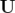and pressure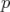, hence the entries for U and p. The keyword relates to a sub-dictionary containing the type of solver and the parameters that the solver uses. The solver is selected through the solver keyword from the options listed below. The parameters, including tolerance, relTol, preconditioner, etc. are described in following sections.

• PCG/PBiCGStab: Stabilised preconditioned (bi-)conjugate gradient, for both symmetric and asymmetric matrices.
• PCG/PBiCG: preconditioned (bi-)conjugate gradient, with PCG for symmetric matrices, PBiCG for asymmetric matrices.
• smoothSolver: solver that uses a smoother.
• GAMG: generalised geometric-algebraic multi-grid.
• diagonal: diagonal solver for explicit systems.

The solvers distinguish between symmetric matrices and asymmetric matrices. The symmetry of the matrix depends on the terms of the equation being solved, e.g. time derivatives and Laplacian terms form coefficients of a symmetric matrix, whereas an advective derivative introduces asymmetry. If the user specifies a symmetric solver for an asymmetric matrix, or vice versa, an error message will be written to advise the user accordingly, e.g.

--> FOAM FATAL IO ERROR : Unknown asymmetric matrix solver PCG
Valid asymmetric matrix solvers are :
3
(
PBiCG
smoothSolver
GAMG
)

#### 4.5.1.1 Solution tolerances

The matrices are sparse, meaning they predominately include coefficients of 0, in segregated, decoupled, finite volume numerics. Consequently, the solvers are generally iterative, i.e. they are based on reducing the equation residual over successive solutions. The residual is ostensibly a measure of the error in the solution so that the smaller it is, the more accurate the solution. More precisely, the residual is evaluated by substituting the current solution into the equation and taking the magnitude of the difference between the left and right hand sides; it is also normalised to make it independent of the scale of the problem being analysed.

Before solving an equation for a particular field, the initial residual is evaluated based on the current values of the field. After each solver iteration the residual is re-evaluated. The solver stops if any one of the following conditions are reached:

• the residual falls below the solver tolerance, tolerance;
• the ratio of current to initial residuals falls below the solver relative tolerance, relTol;
• the number of iterations exceeds a maximum number of iterations, maxIter;

The solver tolerance should represent the level at which the residual is small enough that the solution can be deemed sufficiently accurate. The solver relative tolerance limits the relative improvement from initial to final solution. In transient simulations, it is usual to set the solver relative tolerance to 0 to force the solution to converge to the solver tolerance in each time step. The tolerances, tolerance and relTol must be specified in the dictionaries for all solvers; maxIter is optional and defaults to a value of 1000.

Equations are very often solved multiple times within one solution step, or time step. For example, when using the PISO algorithm, a pressure equation is solved according to the number specified by nCorrectors, as described in section 4.5.3. Where this occurs, the solver is very often set up to use different settings when solving the particular equation for the final time, specified by a keyword that adds Final to the field name. For example, in the cavity tutorial in section 2.1, the solver settings for pressure are as follows.

p
{
solver          PCG;
preconditioner  DIC;
tolerance       1e-06;
relTol          0.05;
}

pFinal
{
\$p;
relTol          0;
}
If the case is specified to solve pressure 4 times within one time step, then the first 3 solutions would use the settings for p with relTol of 0.05, so that the cost of solving each equation is relatively low. Only when the equation is solved the final (4th) time, it solves to a residual level specified by tolerance (since relTol is 0, effectively deactivating it) for greater accuracy, but at greater cost.

#### 4.5.1.2 Preconditioned conjugate gradient solvers

There are a range of options for preconditioning of matrices in the conjugate gradient solvers, represented by the preconditioner keyword in the solver dictionary, listed below. Note that the DIC/DILU preconditioners are exclusively specified in the tutorials in OpenFOAM.

• DIC/DILU: diagonal incomplete-Cholesky (symmetric) and incomplete-LU (asymmetric)
• FDIC: faster diagonal incomplete-Cholesky (DIC with caching, symmetric)
• diagonal: diagonal preconditioning.
• GAMG: geometric-algebraic multi-grid.
• none: no preconditioning.

#### 4.5.1.3 Smooth solvers

The solvers that use a smoother require the choice of smoother to be specified. The smoother options are listed below. The symGaussSeidel and GaussSeidel smoothers are preferred in the tutorials.

• GaussSeidel: Gauss-Seidel.
• symGaussSeidel: symmetric Gauss-Seidel.
• DIC/DILU: diagonal incomplete-Cholesky (symmetric), incomplete-LU (asymmetric).
• DICGaussSeidel: diagonal incomplete-Cholesky/LU with Gauss-Seidel (symmetric/asymmetric).

When using the smooth solvers, the user can optionally specify the number of sweeps, by the nSweeps keyword, before the residual is recalculated. Without setting it, it reverts to a default value of 1.

#### 4.5.1.4 Geometric-algebraic multi-grid solvers

The generalised method of geometric-algebraic multi-grid (GAMG) uses the principle of: generating a quick solution on a mesh with a small number of cells; mapping this solution onto a finer mesh; using it as an initial guess to obtain an accurate solution on the fine mesh. GAMG is faster than standard methods when the increase in speed by solving first on coarser meshes outweighs the additional costs of mesh refinement and mapping of field data. In practice, GAMG starts with the mesh specified by the user and coarsens/refines the mesh in stages. The user is only required to specify an approximate mesh size at the most coarse level in terms of the number of cells

The agglomeration of cells is performed by the method specified by the agglomerator keyword. The tutorials all use the default faceAreaPair method, although the MGridGen option is an alternative method that requires an additional entry specifying the shared object library for MGridGen:

geometricGamgAgglomerationLibs ("libMGridGenGamgAgglomeration.so");
The agglomeration can be controlled using the following optional entries, most of which default in the tutorials.
• cacheAgglomeration: switch specifying caching of the agglomeration strategy (default true).
• nCellsInCoarsestLevel: approximate mesh size at the most coarse level in terms of the number of cells (default 10).
• directSolveCoarset: use a direct solver at the coarsest level (default false).
• mergeLevels: keyword controls the speed at which coarsening or refinement is performed; the default is 1, which is safest, but for simple meshes, the solution speed can be increased by coarsening/refining 2 levels at a time, i.e. setting mergeLevels 2.

Smoothing is specified by the smoother as described in section 4.5.1.3. The number of sweeps used by the smoother at different levels of mesh density are specified by the following optional entries.

• nPreSweeps: number of sweeps as the algorithm is coarsening (default 0).
• preSweepsLevelMultiplier: multiplier for the the number of sweeps between each coarsening level (default 1).
• maxPreSweeps: maximum number of sweeps as the algorithm is coarsening (default 4).
• nPostSweeps: number of sweeps as the algorithm is refining (default 2).
• postSweepsLevelMultiplier: multiplier for the the number of sweeps between each refinement level (default 1).
• maxPostSweeps: maximum number of sweeps as the algorithm is refining (default 4).
• nFinestSweeps: number of sweeps at finest level (default 2).

### 4.5.2 Solution under-relaxation

A second sub-dictionary of fvSolution that is often used in OpenFOAM is relaxationFactors which controls under-relaxation, a technique used for improving stability of a computation, particularly in solving steady-state problems. Under-relaxation works by limiting the amount which a variable changes from one iteration to the next, either by modifying the solution matrix and source prior to solving for a field or by modifying the field directly. An under-relaxation factor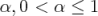specifies the amount of under-relaxation, as described below.

• No specified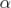: no under-relaxation.
•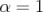: guaranteed matrix diagonal equality/dominance.
•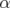decreases, under-relaxation increases.
•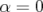: solution does not change with successive iterations.

An optimum choice of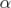is one that is small enough to ensure stable computation but large enough to move the iterative process forward quickly; values of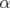as high as 0.9 can ensure stability in some cases and anything much below, say, 0.2 are prohibitively restrictive in slowing the iterative process.

Relaxation factors for under-relaxation of fields are specified within a field sub-dictionary; relaxation factors for equation under-relaxation are within a equations sub-dictionary. An example is shown below from tutorial example of simpleFoam, showing typical settings for an incompressible steady-state solver. The factors are specified for pressure p, pressure U, and turbulent fields grouped using a regular expression.

54
55  relaxationFactors
56  {
57      fields
58      {
59          p               0.3;
60      }
61      equations
62      {
63          U               0.7;
64          "(k|omega|epsilon).*" 0.7;
65      }
66  }
67
68  // ************************************************************************* //

Another example for pimpleFoam, a transient incompressible solver, just uses under-relaxation to ensure matrix diagonal equality, typical of transient simulations.

60
61  relaxationFactors
62  {
63      equations
64      {
65          "U.*"           1;
66          "k.*"           1;
67          "epsilon.*"     1;
68      }
69  }
70
71
72  // ************************************************************************* //

### 4.5.3 PISO, SIMPLE and PIMPLE algorithms

Most fluid dynamics solver applications in OpenFOAM use either the pressure-implicit split-operator (PISO), the semi-implicit method for pressure-linked equations (SIMPLE) algorithms, or a combined PIMPLE algorithm. These algorithms are iterative procedures for coupling equations for momentum and mass conservation, PISO and PIMPLE being used for transient problems and SIMPLE for steady-state.

Within in time, or solution, step, both algorithms solve a pressure equation, to enforce mass conservation, with an explicit correction to velocity to satisfy momentum conservation. They optionally begin each step by solving the momentum equation — the so-called momentum predictor.

While all the algorithms solve the same governing equations (albeit in different forms), the algorithms principally differ in how they loop over the equations. The looping is controlled by input parameters that are listed below. They are set in a dictionary named after the algorithm, i.e. SIMPLE, PISO or PIMPLE.

• nCorrectors: used by PISO, and PIMPLE, sets the number of times the algorithm solves the pressure equation and momentum corrector in each step; typically set to 2 or 3.
• nNonOrthogonalCorrectors: used by all algorithms, specifies repeated solutions of the pressure equation, used to update the explicit non-orthogonal correction, described in section 4.4.4, of the Laplacian term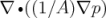; typically set to 0 (particularly for steady-state) or 1.
• nOuterCorrectors: used by PIMPLE, it enables looping over the entire system of equations within on time step, representing the total number of times the system is solved; must be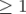and is typically set to 1, replicating the PISO algorithm.
• momentumPredictor: switch that controls solving of the momentum predictor; typically set to off for some flows, including low Reynolds number and multiphase.

### 4.5.4 Pressure referencing

In a closed incompressible system, pressure is relative: it is the pressure range that matters not the absolute values. In these cases, the solver sets a reference level of pRefValue in cell pRefCell. These entries are generally stored in the SIMPLE, PISO or PIMPLE sub-dictionary and are used by those solvers that require them when the case demands it.

### 4.5.5 Other parameters

The fvSolutions dictionaries in the majority of standard OpenFOAM solver applications contain no other entries than those described so far in this section. However, in general the fvSolution dictionary may contain any parameters to control the solvers, algorithms, or in fact anything. If any parameter or sub-dictionary is missing when an solver is run, it will terminate, printing a detailed error message. The user can then add missing parameters accordingly.

OpenFOAM v6 User Guide - 4.5 Solution and algorithm control